# Mandelbrot Fractal

Hi Matthias,

Back to bother you again.
I’ve been assigned a task to make a GDS file of a Mandelbrot Fractal. I’ve found a short VB Excel macro code below.

Sub frac1()
For y = 1 To 200
For x = 1 To 200
i = 0
zx = 0
zy = 0
cx = -2 + x / 50
cy = -2 + y / 50
Do Until (i = 255) Or (zx * zx + zy * zy) >= 4
xt = zx * zy
zx = zx * zx - zy * zy + cx
zy = 2 * xt + cy
i = i + 1
Loop
Cells(y, x).Interior.Color = VBA.RGB(10, 10, i * 10)
‘ my additional line to output X and Y and i values in each cell Cells(y, x).Value = x & "," & y & ",L" & i
Next x
Next y
End Sub

To make a GDS of this I did it the long way in Excel where I got the X and Y co-ordinates and i as the layer then (in excel) defined each layer separately and copied and pasted about 40000 individual “cell.shapes” commands for each individual pixel (1um box).

``````# create a new view (mode 1) with an empty layout
main_window = RBA::Application::instance.main_window
layout = main_window.create_layout(1).layout
layout_view = main_window.current_view

# set the database unit (shown as an example, the default is 0.001)
layout.dbu = 0.001

# create a cell
cell = layout.create_cell("TOP")

# create a layer

Layer1= layout.insert_layer(RBA::LayerInfo::new(1, 0))
Layer2= layout.insert_layer(RBA::LayerInfo::new(2, 0))
Etc  …

cell.shapes(Layer1).insert(RBA::Box::new(29,28,30,29))
cell.shapes(Layer1).insert(RBA::Box::new(30,28,31,29))
cell.shapes(Layer2).insert(RBA::Box::new(31,28,32,29))
cell.shapes(Layer2).insert(RBA::Box::new(32,28,33,29))
etc…  40000 times

# select the top cell in the view, set up the view's layer list and
# fit the viewport to the extensions of our layout
layout_view.select_cell(cell.cell_index, 0)
layout_view.add_missing_layers
layout_view.zoom_fit
``````

It worked but was quite a long process. (see attached image)

I tried using “while” function for the loop but I don’t know how to include the OR statement. I also have trouble defining the layer names i.e. Combining text “Layer” with variable (1) to make Layer1 etc.

Is there any possibility that you could convert the VB routine to Ruby?

Stretching my luck here: Is it also possible to write a Ruby routine that would enable me to merge each layer automatically so that I don’t have to manually view each one individually, select all then merge. i.e. loop that show each layer individually, select all, merge, unselect then move onto next layer.

Many thanks,
Richard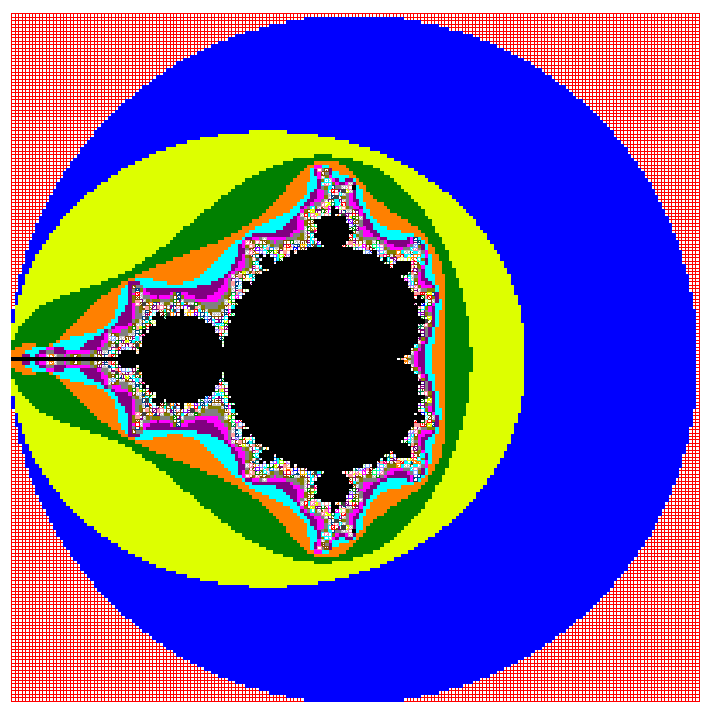## Comments

• Come on, you're pulling my leg, are you?

I bet you guys were sitting together with a beer and tried to come up with the strangest request ever posted to this forum. I'd bet no one ever printed a Mandelbrot set on silicon!

But it's fun, so I give you this:

``````def frac(x, y, nmax)
zx = zy = 0.0
nmax.times do |n|
if zx * zx + zy * zy >= 4.0
return n
end
(zx, zy) = [zx * zx - zy * zy + x, 2 * zx * zy + y]
end
nil
end

nmax = 255

regions = [nil] * nmax

nx = 1000
ny = 1000
pixel_size = 1000

nx.times do |ix|

line_regions = [nil] * nmax

ny.times do |iy|
bx = RBA::Box::new(ix, iy, ix + 1, iy + 1) * pixel_size
f = frac((ix - nx * 0.5) * 4.0 / nx, (iy - ny * 0.5) * 4.0 / ny, nmax)
if f
line_regions[f] ||= RBA::Region::new
line_regions[f].insert(bx)
end
end

line_regions.each_with_index do |r,i|
if r
regions[i] ||= RBA::Region::new
regions[i].insert(r.merged)
end
end

end

ly = RBA::Layout::new
tc = ly.create_cell("TOP")

regions.each_with_index do |r,i|
if r
li = ly.layer(i, 0)
tc.shapes(li).insert(r.merged)
end
end

mw = RBA::MainWindow::instance
mw.create_view
mw.current_view.show_layout(ly, true)
``````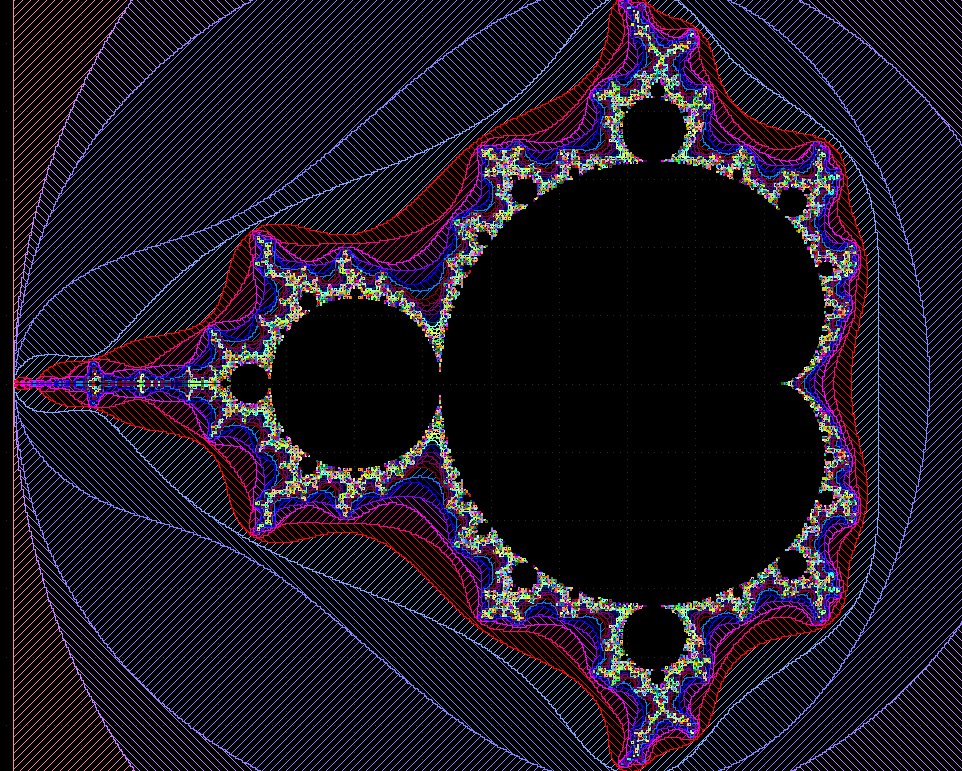Cheers!

Matthias

• Awesome!!!

We're going to try it out on our new e-beam if I can up the resolution and subtract the central (black) region (in the excel code it works if I plot only where i = 255).

Again, I'm in awe of your talent

Very much appreciated

Richard

• This is the most beautiful code I ever seen for ebeamThank you Matthias for sharing the code, I learned a lot from it.

Elias

• Hi Matthias,
Just following up on this, managed to put the design on a photo mask with a 2um pixel. Looks good. Looking forward to the higher definition e-beam write soon.

Cheers
Richard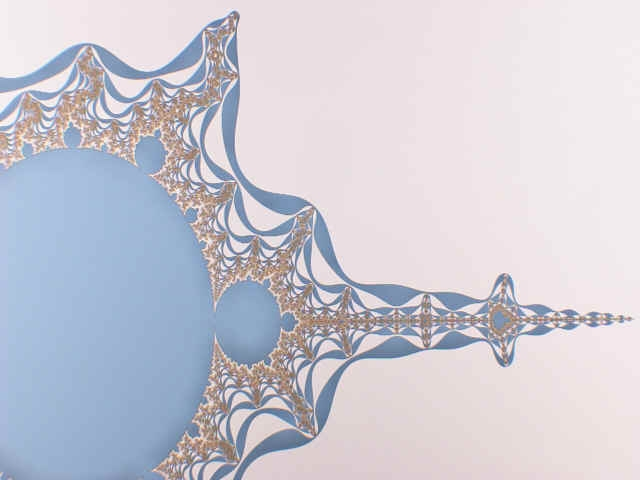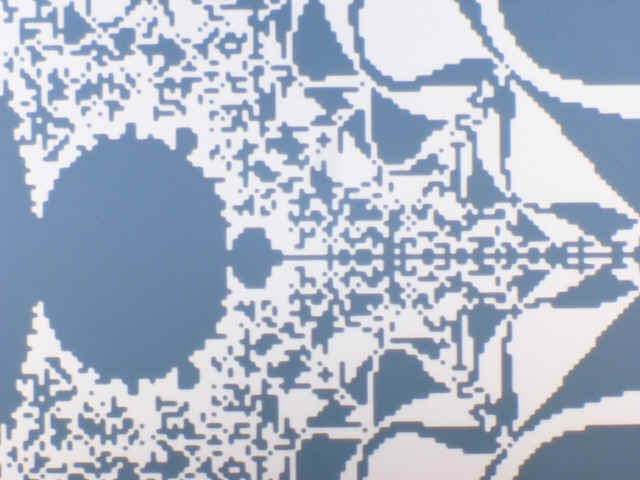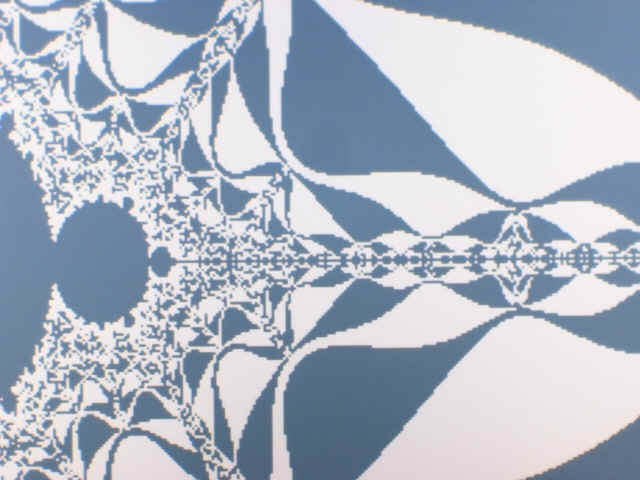• @ram1 Thanks for sharing this nice image!Sign In or Register to comment.